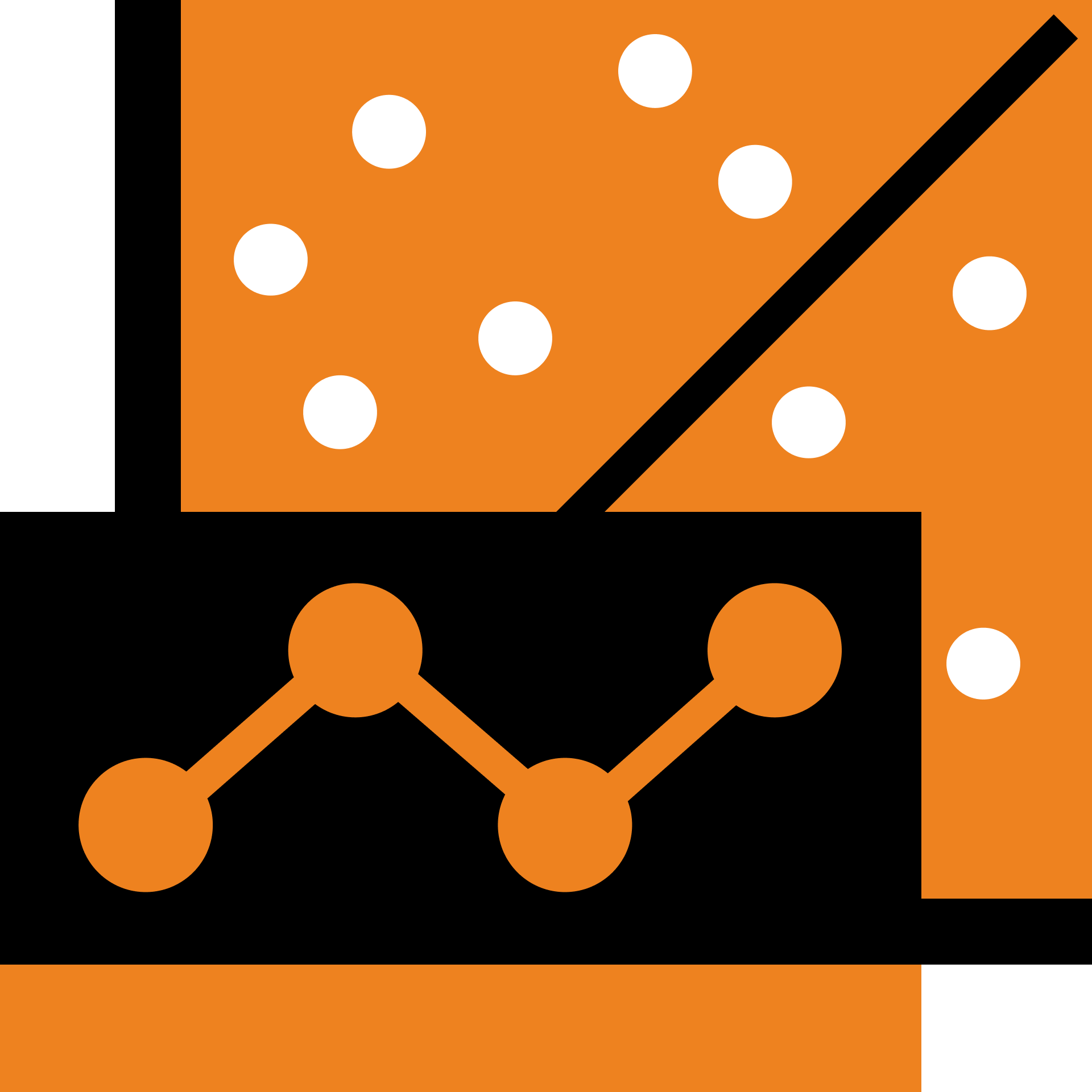## Explaining Variation

Leverage the power of correlation and regression to understand relationships and make predictions with data.

Correlation and Regression

Correlation

Practice Correlation

Correlation Extremes

Practice Correlation Extremes

Simple Linear Regression

Practice Simple Linear Regression

Least Squares

Practice Least Squares

Regression and Prediction

Nonlinear Relationships

### Course description

This course introduces correlation and regression, which are used to quantify the strength of the relationship between variables and to compute the slope and intercept of the regression line. It explores two applications of these methods, using correlated measurements to make informed guesses for measurements that are not available, and making predictions for future events. Datasets used in these lessons include weights and other measurements from penguins and a time series of annual average temperatures. Later lesson explore nonlinear relationships and Simpson's paradox.

### Topics covered

• Correlation
• Regression
• Mean squared error
• Mean absolute error

### Prerequisites and next steps

Exploring Data Visually Introduction to Probability